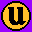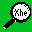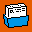# Defining the sort field

The first field of each line starts at 0 (zero); the second is 1 (one) and so on.

To define which field to sort on you give the position of the field at which to start the sort followed by the position at which to end the sort.

The position at which to start the sort is given as the number of fields to skip to get to this position. For example

```   +2
```

tells sort to skip the first two fields.

The position at which to stop the sort is given as the number of the field at the end of which the sort stops. For example

```   -3
```

tells sort to stop the sort at the end of field three.

To sort on the third field of a line use the definition:

```   +2 -3
```

To sort on the fields 5 and 6:

```   +4 -6
```